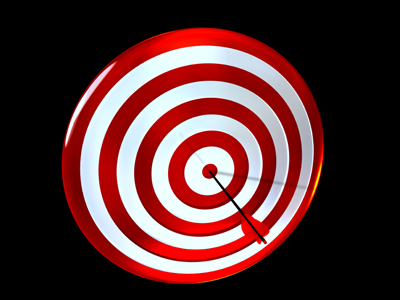The name given to the distance from the center of a polygon or circle to one of the vertices is radius.

# Shapes 2

This Math quiz is called 'Shapes 2' and it has been written by teachers to help you if you are studying the subject at middle school. Playing educational quizzes is a fabulous way to learn if you are in the 6th, 7th or 8th grade - aged 11 to 14.

It costs only \$12.50 per month to play this quiz and over 3,500 others that help you with your school work. You can subscribe on the page at Join Us

Rectangles and squares are 2D shapes, or polygons. Cuboids and cubes are 3D shapes, or polyhedrons. There are many words used to describe polygons and polyhedrons, such as angles, radius and circumference. But what do these words mean? You will have learned about them in your classes; now test how much you have remembered.

Enjoy playing this quiz on shapes.

1.
What is the name given to the distance from the center of a polygon or circle to one of the vertices?
Circumference
Diameter
Tangent
The diameter is twice the radius
2.
How many degrees in a right angle?
60o
90o
180o
360o
There are 360o in a full turn so a quarter turn will be 90o
3.
What is a seven sided polygon called?
Heptagon
Hexagon
Pentagon
Tetrahedron
A heptagon has 7 sides and a heptagram is a star with 7 points
4.
What is the name of the triangle with all sides and all angles equal?
Right angled triangle
Isosceles triangle
Equilateral triangle
Pyramid
An equilateral triangle has angles of 60o at each of its corners
5.
Which of these describes an isosceles triangle?
Triangle with 3 equal sides
Triangle with 2 equal sides and 2 equal angles
Triangle with 3 equal sides and 2 equal angles
Triangle with 2 equal sides and 3 equal angles
An isosceles triangle has 2 equal angles which are opposite to 2 sides of equal length
6.
What is a polyhedron?
A 3D shape where all faces are square
A 3D shape where all faces are flat polygons
A 2D shape where all angles are different
A 2D shape with twelve sides
Cubes and cuboids are both polyhedrons
7.
How many sides does an octagon have?
5
6
7
8
To help remember this, think of an octopus with 8 legs
8.
Which of these is a regular polygon?
Rectangle
Right angled triangle
Square
Circle
A regular polygon has all sides equal
9.
What is the name of all flat shapes with three or more straight sides?
Triangle
Polyhedron
Polygon
Heptagon
So triangles, squares, rectangles and pentagons are all polygons
10.
What is the name given to a shape that has four faces all of which are equilateral triangles?
Tetrahedron
Polyhedron
Prism
Pyramid
Tetrahedrons are similar to pyramids, but pyramids have 5 faces, one of which is a square
Author:  Amanda Swift